## 3.9 Special Rules and Scales

The above sections describe most of the basic scales that can be found on typical slide rules. Many other specialty rules also were developed for particular types of calculations found in various professions. For example, an “Electro” slide rule is a type that was made by various manufacturers for use in the electrical trades. It has Gauge Marks on certain scales corresponding to the resistivity of copper, assuming diameters of wire in inches or millimeters, for instance, and lengths in feet or meters. Knowing the standard voltage used in the system, voltage drops, currents, and power levels can be quickly computed.

There were “chemical” slide rules used in the petroleum industry with built-in marks indicating commonly used densities and other properties of various compounds; “radio” and “electronics” rules used in the radio and electrical engineering industries with special scales for relationships between frequencies, capacitance, decibels, and other common variables; stadia rules used in surveying, which provided direct calculations of horizontal offset and vertical rise from transit readings; “artillery” rules used in the military; “proportions” rules used in typography and engraving applications, and so forth.

Another special type of slide rule was one that provided scales of the hyperbolic trigonometric functions. These hyperbolic sine (Sh, or SH) and hyperbolic tangent (Th, or TH) scales can be used to performed what are called vector calculations and hence rules with these scales are often called Vector rules. A discussion of these special scales and their use are described in the vignette Vector/Hyperbolic Calculations.

In many cases these special rules can be quite rare and are highly sought after by collectors. (Personally, I’d love to have a Black Body radiation slide rule!)

$$~$$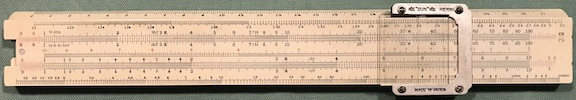Hemmi Electro.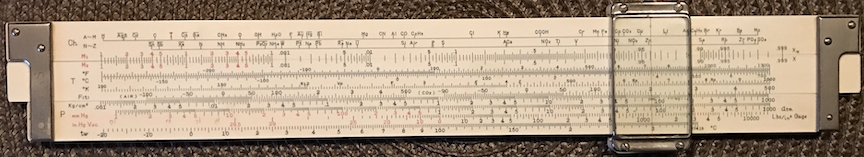Hemmi Chemical Rule.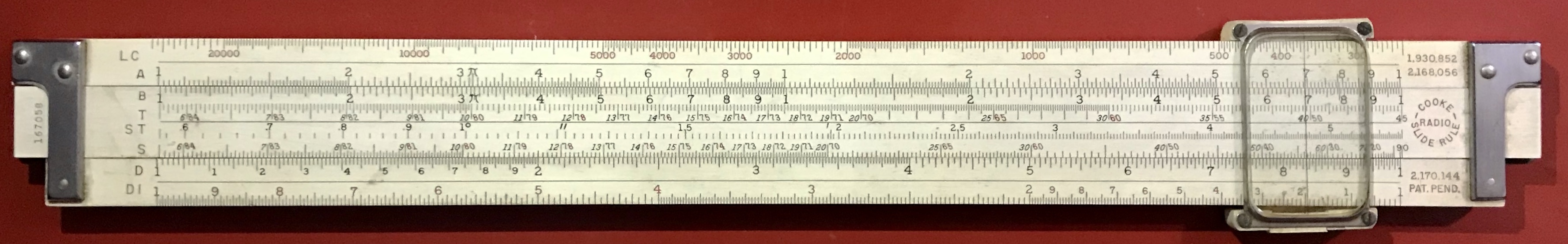Pickett Model N-515-T Electronics Rule.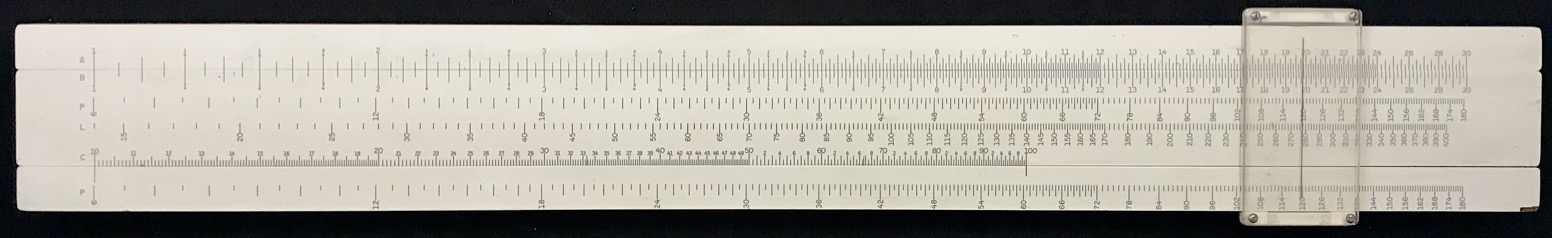Engraving Rule.K&E Model 4083-3 Vector Rule (back).

Lastly, there are rules with very long scales for reading values to many more significant figures. Some of these are themselves quite long – 20 inch Mannheim-type rules were common in the early part of the 20th century. Circular rules also can give higher accuracy with scales that can be up to a factor of $$\pi$$ longer than their overall dimension. Spiral scales (such as on the Boykin RotaRule) and even helical scales (such as the Otis King’s Calculator and the Fuller Calculator) can give total scale lengths of up to several feet, providing multiplications and division with impressive accuracy. Examples can be found in the section Non-Linear and Long-Scale Rules.

Some of the more modern rules have certain scales that are broken up into segments, so that a 20-inch scale can fit onto a 10-inch rule, for instance. One of my favorites is the Pickett Model 4. (See figure below.) It has the following scales on it:

Front:

• a 30-inch equivalent cube/cube-root scale
• a 20-inch equivalent square/square-root scale
• 20-inch equivalent T and S/ST scales
• standard 10-inch C/D, CI/DI, CF/DF scales

Back:

• standard 10-inch C/D, CI scales
• CFm/DFm scales – folded at value of $$\ln 10$$ for conversion between Base $$e$$ and Base 10
• 80-inch equivalent log-log scale
• TH and SH scales for direct reading of hyperbolic trigonometric functions and direct vector calculations

And all in Eye-Saver yellow with black text!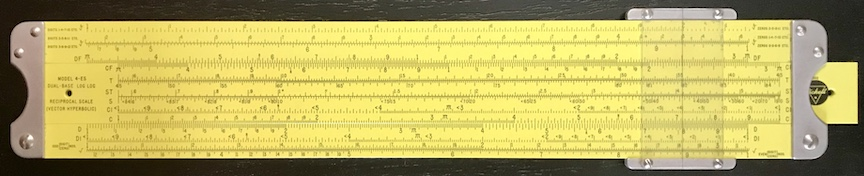Pickett Model 4-ES (front).Pickett Model 4-ES (back).